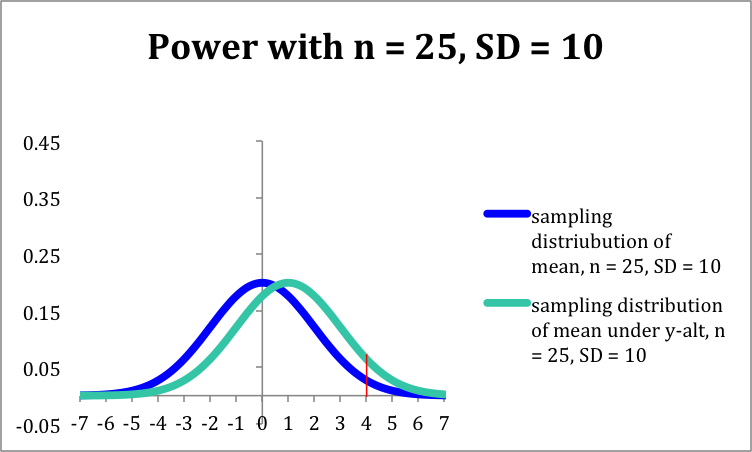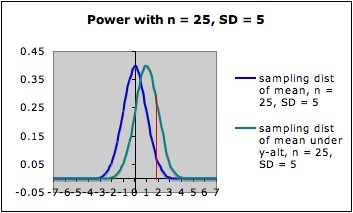COMMON MISTEAKS MISTAKES IN USING STATISTICS: Spotting and Avoiding Them

## Factors that Affect the Power of a Statistical Procedure

As discussed on the page Power of a Statistical Procedure, the power of a statistical procedure depends on the specific alternative chosen (for a hypothesis test) or a similar specification, such as width of confidence interval (for a confidence interval).

The following factors also influence power:

1. Sample Size

Power depends on sample size. Other things being equal, larger sample size yields higher power. Example and more details.

2. Variance

Power also depends on variance: smaller variance yields higher power.

Example: The pictures below each show the sampling distribution for the mean under the null hypothesis µ = 0 (blue -- on the left in each picture) together with the sampling distribution under the alternate hypothesis µ = 1 (green -- on the right in each picture), both with sample size 25, but for different standard deviations of the underlying distributions. (Different standard deviations might arise from using two different measuring instruments, or from considering two different populations.)
• In the first picture, the standard deviation is 10; in the second picture, it is 5.
• Note that both graphs are in the same scale. In both pictures, the blue curve is centered at 0 (corresponding to the the null hypothesis) and the green curve is centered at 1 (corresponding to the alternate hypothesis).
• In each picture, the red line is the cut-off for rejection with alpha = 0.05 (for a one-tailed test) -- that is, in each picture, the area under the blue curve to the right of the red line is 0.05.
• In each picture, the area under the green curve to the right of the red line is the power of the test against the alternate depicted. Note that this area is larger in the second picture (the one with smaller standard deviation) than in the first picture.The Claremont University's Wise Project's Statistical Power Applet and the Rice Virtual Lab in Statistics' Robustness Simulation can be used to illustrate this dependence in an interactive manner.

Variance can sometimes be reduced by using a better measuring instrument, restricting to a subpopulation, or by choosing a better experimental design (see below).

3. Experimental Design

Power can sometimes be increased by adopting a different experimental design that has lower error variance. For example, stratified sampling or blocking can often reduce error variance and hence increase power. However,

For more on designs that may increase power, see:
• Lipsey, MW (1990). Design sensitivity: Statistical power for experimental research. Newbury Park, CA: Sage.
• McClelland, Gary H. (2000) Increasing statistical power without increasing sample size, American Psychologist 55(8), 963 - 964
Last updated June 2012